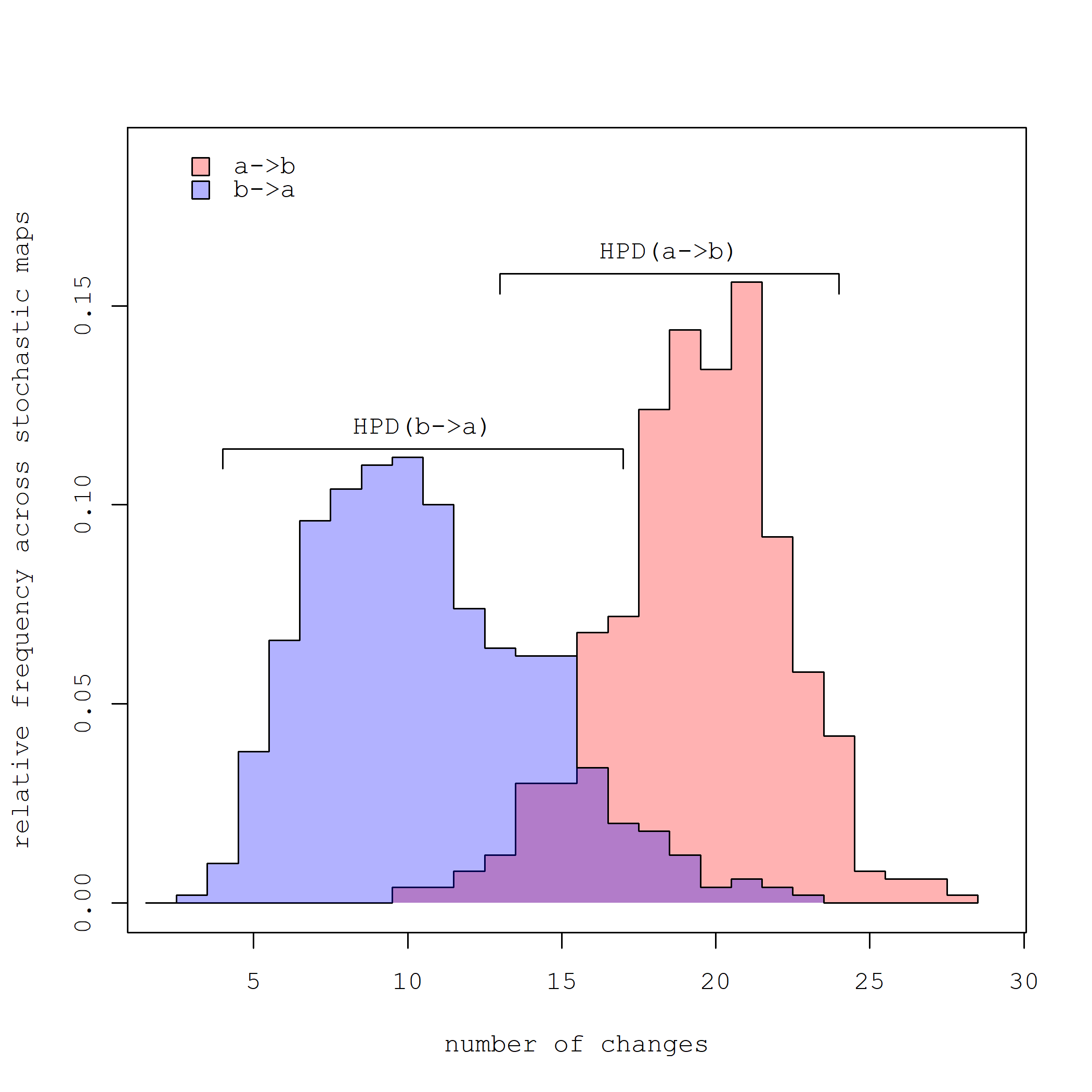## Monday, June 3, 2019

### Overlooked phytools function: S3 `density` method for `"multiSimmap"` object class

Among the list of phytools functions that may sometimes be overlooked, I'd like to mention the simple but neat `density` S3 method for the phytools object class `"multiSimmap"`.

The function computes (and an associated `plot` method graphs) a posterior density on the number of changes between states for the character across our sample of stochastic character maps. The following is a short demo of how that looks.

First, here are some data & a tree:

``````library(phytools)
tree
``````
``````##
## Phylogenetic tree with 150 tips and 149 internal nodes.
##
## Tip labels:
##  t23, t50, t51, t72, t73, t102, ...
##
## Rooted; includes branch lengths.
``````
``````x
``````
``````##  t23  t50  t51  t72  t73 t102 t103  t93  t64  t94  t95  t11  t19  t89  t90
##    a    a    a    a    a    a    a    a    a    a    a    b    a    a    a
##   t3  t54  t55  t56  t81  t82  t27  t57  t91  t92  t38  t39  t37  t47 t145
##    a    a    a    a    b    b    b    b    a    b    b    b    b    b    b
## t146   t2  t24  t58  t59  t60  t61 t123 t124   t6  t42  t43 t100 t101  t16
##    b    b    a    a    a    a    a    b    b    a    a    b    a    b    a
##   t8   t9   t7  t74  t75  t69 t143 t144  t41 t132 t133   t1 t129 t130  t35
##    a    b    a    a    a    a    b    b    a    b    b    b    b    b    b
##  t36  t31  t65 t119 t120 t118  t87  t52  t53 t108 t109 t141 t142  t20  t21
##    b    b    b    a    b    b    b    b    b    a    a    b    b    a    b
##  t25  t26  t45  t46 t104 t105  t98  t99  t30  t83  t84 t138 t139 t106 t107
##    a    a    a    a    a    a    a    a    a    a    a    a    a    a    a
##  t85  t86  t71  t12  t67  t68  t62  t63  t28  t29  t33  t34 t136 t137 t125
##    a    a    b    a    a    a    a    a    b    b    a    a    b    b    a
## t126  t10   t4   t5  t17  t18 t114 t115  t22 t127 t128  t96  t97  t70 t147
##    a    a    b    a    a    a    a    a    a    a    a    a    a    a    a
## t148 t131  t48  t32  t79  t80  t66 t116 t117  t88 t112 t113  t49 t121 t122
##    a    a    a    a    a    a    b    b    b    b    b    b    b    b    b
##  t44  t76  t77 t110 t111  t13  t14 t140 t149 t150  t78 t134 t135  t40  t15
##    b    b    b    b    b    b    b    a    a    a    a    a    a    a    b
## Levels: a b
``````

Now, let's undertake our stochastic mapping:

``````mtrees<-make.simmap(tree,x,nsim=500,model="ARD")
``````
``````## make.simmap is sampling character histories conditioned on the transition matrix
##
## Q =
##            a          b
## a -0.8282081  0.8282081
## b  0.9020183 -0.9020183
## (estimated using likelihood);
## and (mean) root node prior probabilities
## pi =
##   a   b
## 0.5 0.5
``````
``````## Done.
``````
``````mtrees
``````
``````## 500 phylogenetic trees with mapped discrete characters
``````

Finally, we'll use our `density` method:

``````d<-density(mtrees)
d
``````
``````##
## Distribution of changes from stochastic mapping:
##  a->b        b->a
##  Min.   :10  Min.   :3
##  Median :20  Median :10
##  Mean   :19.38   Mean   :10.64
##  Max.   :28  Max.   :23
##
## 95% HPD interval(a->b): [13, 24]
## 95% HPD interval(b->a): [4, 17]
``````
``````par(family="mono")
plot(d)
``````Just for interest, the tree & data were simulated as follows:

``````library(phytools)
Q<-matrix(c(-0.8,0.8,0.4,-0.4),2,2,byrow=TRUE)
rownames(Q)<-colnames(Q)<-letters[1:2]
tree<-pbtree(n=150,scale=1)
x<-sim.Mk(tree,Q)
``````